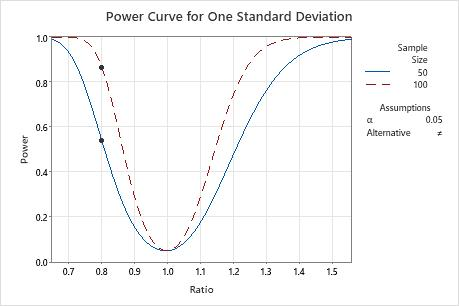# Example of Power and Sample Size for 1 Variance

The manager of a lumber yard wants to assess the performance of a saw mill that cuts beams that are supposed to be 100 cm long. The manager plans to perform a 1 variance test to determine the variability of the mill.

Before collecting the data for a 1 variance test, the manager uses a power and sample size calculation to determine what the power of the test will be when the sample sizes are 50 and 100 and the test detects a 0.8 ratio between the comparison and hypothesized standard deviations.

1. Choose Stat > Power and Sample Size > 1 Variance.
2. In Sample sizes, enter 50 100.
3. In Ratios, enter 0.8.
4. Click OK.

## Interpret the results

To detect a ratio of 0.8, the analyst can obtain a power of 0.539 with a sample size of 50 and a power of 0.865 with a sample size of 100. To have enough power to detect a ratio of 0.8, the analyst decides to collect 100 samples.

### Power and Sample Size

Test for One Standard Deviation Testing StDev = null (versus ≠ null) Calculating power for (StDev / null) = ratio α = 0.05
Results Sample Ratio Size Power 0.8 50 0.539065 0.8 100 0.865153

### Power Curve for One Standard DeviationBy using this site you agree to the use of cookies for analytics and personalized content.  Read our policy